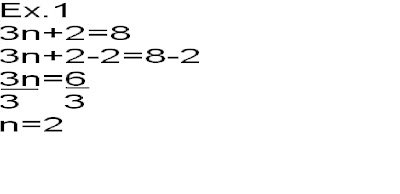## Tuesday, May 10, 2011

### Alec's Algebra Post

Solving One Step Algebra Equation
1. Isolate Variable
2.Cancel constant using the opposite integer
3.Balance it out by doing the same thing on the other side
4.Verify- solve to equalitySolving the question using Alge TilesSolving Two Step Algebra Equation
1. Isolate the variable (the unknown number in an algebra question)
2. Cancel the constant (the integer in an algebra question)
3. Balance by doing the same thing on one side and the other side of the equal sign
4. Simplify the variable
5. Repeat step #3